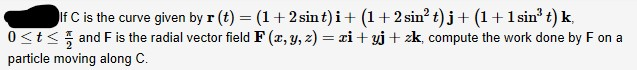# If C is the curve given by r (t) = (1+2 sint)i+ (1+ 2 sin? t)j+ (1+1 sin' t) k,0

Question
2 viewshelp_outlineImage TranscriptioncloseIf C is the curve given by r (t) = (1+2 sint)i+ (1+ 2 sin? t)j+ (1+1 sin' t) k, 0
check_circle

Step 1

Given:

Step 2

Compute the derivative of r(t) as shown below.

Step 3

The work done is obtained ...

### Want to see the full answer?

See Solution

#### Want to see this answer and more?

Solutions are written by subject experts who are available 24/7. Questions are typically answered within 1 hour.*

See Solution
*Response times may vary by subject and question.
Tagged in

### Other